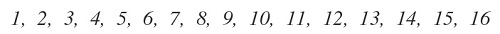# Distributing numbersGiven the distribution of numbers shown above, it is requested:

• Distribute the numbers in two groups A and B of 8 numbers each.
• The sum of all the numbers in group A must equal the sum of all the numbers in group B.
• The sum of all the squares of the numbers in group A must be equal to the sum of all the squares in group B.

Is it possible to satisfy the requested conditions for the distribution of numbers?

×